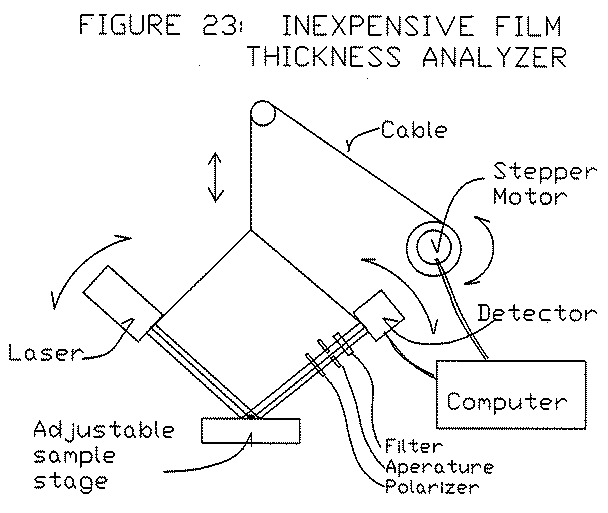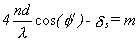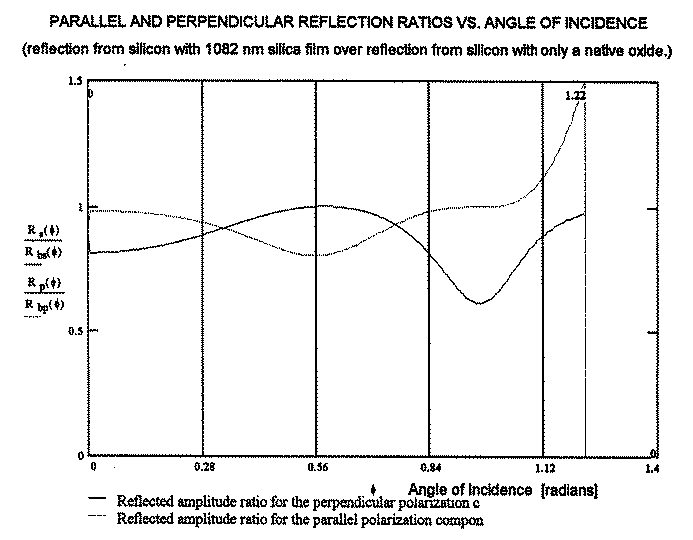﻿ Spectrometry and Ellipsometry Analysis -- Redeeming Time DesignsTHIN FILM ANALYSIS USING SPECTROMETRY AND ELLIPSOMETRY

Objective #4:

IV. 1  Inexpensive and Versatile Film Measurement Device:

The polarized spectrophotometer described in Objectives #2 is very powerful because so much information can be generated.  However, it requires the use of an expensive spectrophotometer.  Therefore, the question becomes, can an inexpensive machine be designed to perform like this equipment and possess as much of the positive qualities of Table IX?  Such a design is proposed in Figure 23, where the incident angle of a laser beam is varied from a small angle to about the polarization angle.  A MathCad program was written to generate a theoretical curve that would be generated by this equipment if the silicon dioxide covered wafer were analyzed.FIGURE 23:  Inexpensive Film Thickness Analyzer.

The MathCad program and Figure 24 shows the theoretical curve generated.  In Figure 24, there is a pair of a maximum and a minimum for the two s- and p- components and there is a s-component minimum, unpaired due to the polarization angle.  Therefore, it can be immediately seen that both n and d can easily be determined independently, by Equation 3.(3)

For instance, Equation 3 can be determined at the maximum and at the minimum shown in Figure 24 for s-component, for two consecutive m integer values.  Unlike spectrophotometry, n remains constant as φ varies, if the film is assumed totally transparent.  Also, δs is a function of φ, which allow the n and d to be separated from the n*d term at each point.  The complex index of the substrate does vary with φ, but it varies by a relation which is independent of material.  The relation is included in the MathCad program.FIGURE 24:  Parallel and Perpendicular Reflection Ratios versus Angle of Incidence.  (Reflected from silicon with 1082 nm silica film over reflection from silicon with only a native oxide.)

Home